DraftKings 2019 Championship Odds
+240
2.4 to 1Bucks
23.0% implied probability

+300
3 to 1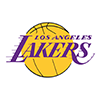Lakers
19.5% implied probability

+330
3.3 to 1Clippers
18.1% implied probability

+1200
12 to 1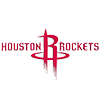Rockets
6.0% implied probability

+1400
14 to 176ers
5.2% implied probability

+1800
18 to 1Jazz
4.1% implied probability

+2000
20 to 1Raptors
3.7% implied probability

+2000
20 to 1Celtics
3.7% implied probability

+2000
20 to 1Nuggets
3.7% implied probability

+2000
20 to 1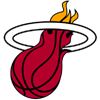Heat
3.7% implied probability

+3300
33 to 1Mavericks
2.3% implied probability

+6600
66 to 1Pacers
1.2% implied probability

+10000
100 to 1Trail Blazers
0.8% implied probability

+10000
100 to 1Nets
0.8% implied probability

+15000
150 to 1Thunder
0.5% implied probability

+15000
150 to 1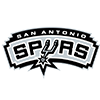Spurs
0.5% implied probability

+20000
200 to 1Grizzlies
0.4% implied probability

+25000
250 to 1Magic
0.3% implied probability

+25000
250 to 1Timberwolves
0.3% implied probability

+25000
250 to 1Suns
0.3% implied probability

+25000
250 to 1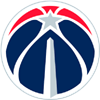Wizards
0.3% implied probability

+25000
250 to 1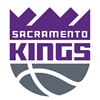Kings
0.3% implied probability

+25000
250 to 1Pelicans
0.3% implied probability

+50000
500 to 1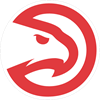Hawks
0.2% implied probability

+50000
500 to 1Pistons
0.2% implied probability

+50000
500 to 1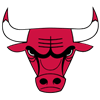Bulls
0.2% implied probability

+50000
500 to 1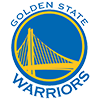Warriors
0.2% implied probability

+80000
800 to 1Cavaliers
0.1% implied probability

+80000
800 to 1Hornets
0.1% implied probability

+80000
800 to 1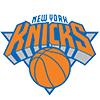Knicks
0.1% implied probability